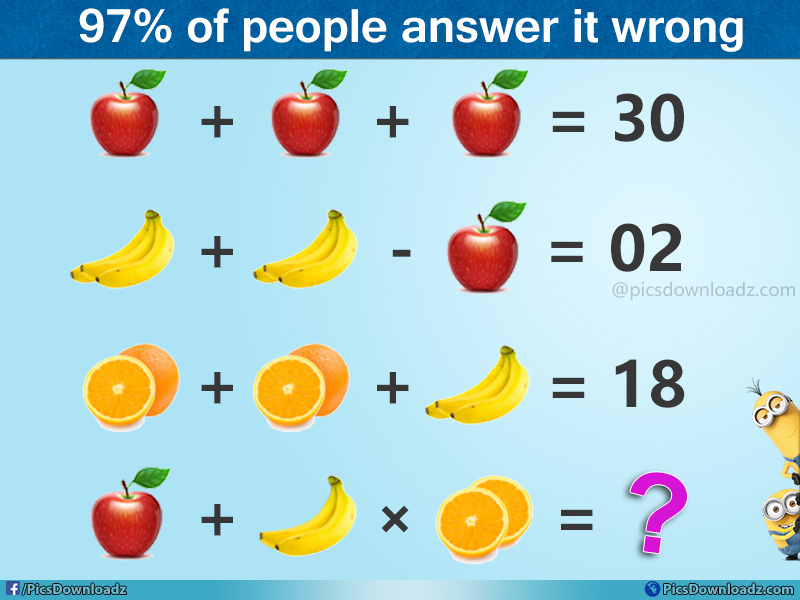This post may contain affiliate links. This means if you click on the link and purchase the item, We will receive an affiliate commission at no extra cost to you. See Our Affiliate Policy for more info.

Apple + Banana × Orange = ?? – The Viral Fruits Math Puzzle with Answer

0
7953

97% of people answer it wrong. Solve this genius fruit math puzzle. The most viral apple, banana, and orange puzzle only for genius with the answer.

Hello, Friends! solve this interesting viral apple, banana fruits math puzzle. Find the value of apple, banana, and orange, and then solve the last equation. One of the best tricky math puzzle image. Let see how many of you can find the correct answer.

Share your answer in the comment section below before you confirm it. Good luck.

Puzzle Question:

⇒ Apple + Apple + Apple = 30

⇒ Banana + Banana – Apple = 02

⇒ Orange + Orange + Banana = 18

⇒ Apple + Banana × Orange = ??

Viral Fruits Math Puzzle Image:[Embed Code for this Image given below]

.

A

N

S

W

E

R

.

Equation 1:

⇒ Apple + Apple + Apple = 30

⇒ 3 Apple = 30

⇒ Apple = 30/3

⇒ Apple = 10

Equation 2:

⇒ Banana + Banana – Apple = 02

⇒ 2 Banana = 02 + Apple

⇒ 2 Banana = 02 + 10

⇒ 2 Banana = 12

⇒ Banana = 12/2

⇒ Banana = 6

Equation 3:

⇒ Orange + Orange + Banana = 18

⇒ 2 Orange = 18 – Banana

⇒ 2 Orange = 18 – 6

⇒ 2 Orange = 12

⇒ Orange = 12/2

⇒ Orange = 6

Equation 4:

Trick Part:

If you noticed there are only two bananas in the last equation as compare to third equation which has three bananas. Also One + Half Orange in third equation but in last equation both oranges are half.

Previous Equation vs Last Equation

⇒ Three Banana vs Two Banana in last Equation

⇒ (One + Half) Orange vs (Half + Half) Orange in last equation

Therefore:

From Second Equation;

⇒ Banana (3) = 6,

So,

⇒ One Banana = 6/3 = 2

⇒ Two Bananas = 2+2 = 4

Also From Third Equation;

⇒ (One + Half) Orange = 6

⇒ One Orange + Half Orange = 6

⇒ One Orange = 4

⇒ & Half Orange = 2

Now Last Equation become;

⇒ Apple + Banana (Two) × Orange (Half + Half) = ??

• Apple = 10
• Bananas (Two) = 2+2 = 4
• Orange (Half + Half) = 2+2 = 4

Therefore:

⇒ 10 + 4 × 4 = ?? (Acc. to order of operation, multiplication first)

⇒ 10 + 16 = 26 Correct Answer

Hope, you found this puzzle interesting. 😉

LIKE | SHARE | PIN IT

Share this genius puzzle in your group let see how many of them can solve this puzzle. Like our fb page and join our puzzle group for more interesting puzzle image. Enjoy Take Care!

Like our Page: